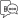Explore Categories

# Maths Related Functions

## \$\$RandomNumber

A random number is a number generated by a process whose outcome is unpredictable, and which cannot be subsequently reliably reproduced. In other words, Random numbers are numbers that occur in a sequence such that, the values are uniformly distributed over a defined interval and it is impossible to predict future values based on past or present ones.

In this release, a new TDL function \$\$RandomNumber has been introduced to generate Random Numbers. In case of auditing, this can be useful for auditors who would like to pick up some vouchers randomly for authentication.

Syntax

\$\$RandomNumber[:<MinRange>[:<MaxRange>]]

Where,

<Min Range> and <Max Range> are optional. In the absence of Max Range, Long Max is considered, i.e., (2^31) – 1 = 2147483647. In the absence of Min Range, ZERO(0) is considered.

We can generate random numbers in different ways:

No Parameters: Don’t pass any parameters, i.e., just invoke \$\$ RandomNumber . Default values are assumed.

Only with the MinRange Parameter: Here, there is no need of passing Max range. In this scenario, Random number is generated from the given Min Range.

Both MaxRange and MinRange as Parameter: In this scenario, random numbers are generated for given range.

Example: 1

With no Parameters

Set As : \$\$RandomNumber

This code will return a Random Number between 0 and 2147483647.

Example: 2

With MinRange Parameter only

Set As : \$\$RandomNumber:9999

This code returns Random Numbers between 9999 and 2147483647, the random number being greater than or equal to 9999. Here, value of MinRange is 9999 and MaxRange is 2147483647.

Example: 3

With both Parameters (MinRange and MaxRange)

Set As : \$\$RandomNumber:9:9999

This code returns Random Numbers between 9 and 9999.Helpful?